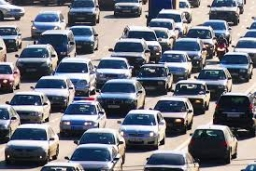# Travels 20483

When and where will the two cars that drove at the same time from cities A and B 90 km apart if the car from city A travels at a speed of 75 km/h and the car from city B at a speed of 60 km/h meet?

t =  0.6667 h
s1 =  50 km

### Step-by-step explanation:Did you find an error or inaccuracy? Feel free to write us. Thank you!

Tips for related online calculators
Do you have a linear equation or system of equations and looking for its solution? Or do you have a quadratic equation?
Do you want to convert velocity (speed) units?
Do you want to convert time units like minutes to seconds?